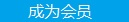您可以捐助，支持我们的公益事业。 1元 10元 50元 认证码：必填求知 文章 文库 Lib 视频 iProcess 课程 认证 咨询 工具 讲座 Modeler Code要资料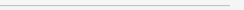订阅捐助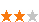1224 次浏览     评价： 好 中 差
2018-11-21

 编辑推荐: 本文来自于csdn，本文章主要对真实数据（csv表格数据）的查看以及如何正确的分开训练测试集进行实战操作，希望对您的学习有帮助。

５、查看训练集的特征图像信息以及特征之间的相关性

 train_housing = strat_train_set.copy() train_housing.plot(kind="scatter", x="longitude", y="latitude")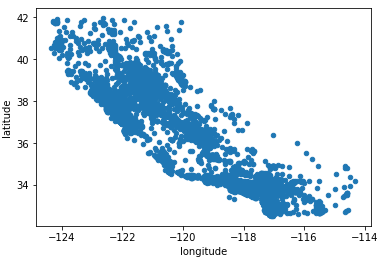train_housing.plot(kind="scatter", x="longitude", y="latitude", alpha=0.1)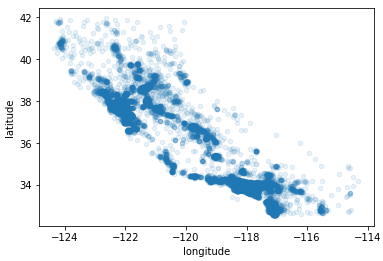train_housing.plot(kind="scatter", x="longitude", y="latitude", alpha=0.4, s=housing["population"]/100, label="population", c="median_house_value", cmap=plt.get_cmap("jet"), colorbar=True,) plt.legend()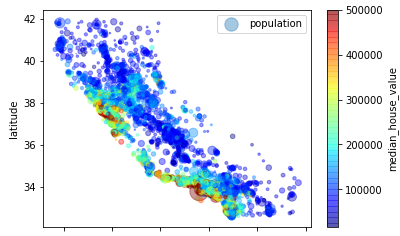corr_matrix = train_housing.corr() corr_matrix["median_house_value"].sort_values (ascending=False)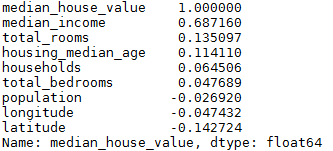from pandas.tools.plotting import scatter_matrix attributes = ["median_house_value", "median_income", "total_rooms"] scatter_matrix(housing[attributes], figsize=(12, 8))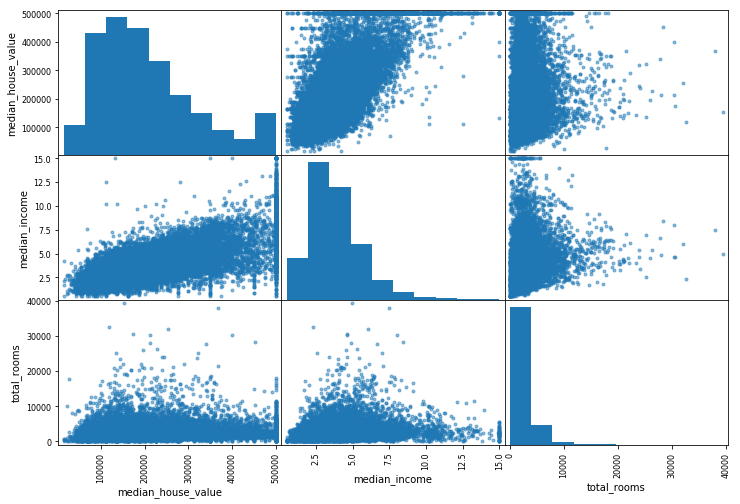train_housing["rooms_per_household"] = housing ["total_rooms"]/housing["households"] train_housing["bedrooms_per_room"] = housing ["total_bedrooms"]/housing["total_rooms"] train_housing["population_per_household"]=housing ["population"]/housing["households"] corr_matrix = train_housing.corr() corr_matrix["median_house_value"].sort_values (ascending=False)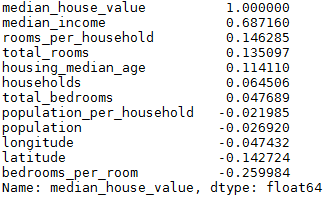6、准备数据（数据预处理）

 train_housing = strat_train_set.drop("median_house_value", axis=1) train_housing_labels = strat_train_set["median_house_value"].copy()

1、去掉含有缺失值的个体（dropna）

2、去掉含有缺失值的整个特征（drop）

3、给缺失值补上一些值（0、平均数、中位数等）（fillna）

 #train_housing.dropna(subset=["total_bedrooms"]) # option 1 #train_housing.drop("total_bedrooms", axis=1) # option 2 median = train_housing["total_bedrooms"].median() train_housing["total_bedrooms"].fillna(median) # option 3

 from sklearn.preprocessing import Imputer imputer = Imputer(strategy="median") housing_num = train_housing.drop("ocean_proximity", axis=1) imputer.fit(housing_num)

 X = imputer.transform(housing_num) #X = imputer.fit_transform(housing_num) #也可以将numpy格式的转换为pd格式 #housing_tr = pd.DataFrame(X, columns=housing_num.columns)

 from sklearn.preprocessing import LabelEncoder encoder = LabelEncoder() housing_cat = train_housing["ocean_proximity"] housing_cat_encoded = encoder.fit_transform(housing_cat) print(housing_cat_encoded) print(encoder.classes_)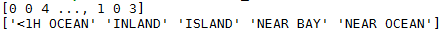from sklearn.preprocessing import OneHotEncoder encoder = OneHotEncoder() housing_cat_1hot = encoder.fit_transform(housing_cat_encoded.reshape(-1,1))

 housing_cat_1hot.toarray()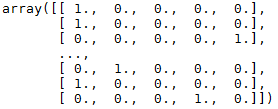from sklearn.preprocessing import LabelBinarizer encoder = LabelBinarizer() #encoder = LabelBinarizer(sparse_output=True) housing_cat_1hot = encoder.fit_transform(housing_cat)

 from sklearn.base import BaseEstimator, TransformerMixin rooms_ix, bedrooms_ix, population_ix, household_ix = 3, 4, 5, 6 class CombinedAttributesAdder(BaseEstimator, TransformerMixin): def __init__(self, add_bedrooms_per_room = True): # no *args or **kargs self.add_bedrooms_per_room = add_bedrooms_per_room def fit(self, X, y=None): return self # nothing else to do def transform(self, X, y=None): rooms_per_household = X[:, rooms_ix] / X[:, household_ix] population_per_household = X[:, population_ix] / X[:, household_ix] if self.add_bedrooms_per_room: bedrooms_per_room = X[:, bedrooms_ix] / X[:, rooms_ix] return np.c_[X, rooms_per_household, population_per_household, bedrooms_per_room] else: return np.c_[X, rooms_per_household, population_per_household] attr_adder = CombinedAttributesAdder(add_bedrooms_per_room=False) housing_extra_attribs = attr_adder.transform(housing.values)

1、MinMaxScaler：将特征缩放到0-1之间，但异常值对这个影响比较大，比如异常值为100，缩放0-15为0-0.15;

2、feature_range：可以自定义缩放的范围，不一定是0-1;

3、StandardScaler：标准化（减均值，除方差），对异常值的影响较小，但可能会不符合某种范围

Transformation Pipelines

 from sklearn.pipeline import Pipeline from sklearn.preprocessing import StandardScaler num_pipeline = Pipeline([ ('imputer', Imputer(strategy="median")), ('attribs_adder', CombinedAttributesAdder()), ('std_scaler', StandardScaler()), ]) housing_num_tr = num_pipeline.fit_transform(housing_num)

Pipeline是由（name(名字)，Estimator(类)）对组成，但最后一个必须为transformer，这是因为要形成fit_transform()方法

 from sklearn.base import BaseEstimator, TransformerMixin class DataFrameSelector(BaseEstimator, TransformerMixin): def __init__(self, attribute_names): self.attribute_names = attribute_names def fit(self, X, y=None): return self def transform(self, X): return X[self.attribute_names].values

 from sklearn.pipeline import FeatureUnion num_attribs = list(housing_num) cat_attribs = ["ocean_proximity"] num_pipeline = Pipeline([ ('selector', DataFrameSelector(num_attribs)), ('imputer', Imputer(strategy="median")), ('attribs_adder', CombinedAttributesAdder()), ('std_scaler', StandardScaler()), ]) cat_pipeline = Pipeline([ ('selector', DataFrameSelector(cat_attribs)), ('label_binarizer', LabelBinarizer()), ]) full_pipeline = FeatureUnion(transformer_list=[ ("num_pipeline", num_pipeline), ("cat_pipeline", cat_pipeline), ]) housing_prepared = full_pipeline.fit_transform(train_housing) ！

 from sklearn.base import BaseEstimator, TransformerMixin class LabelBinarizer_new(TransformerMixin, BaseEstimator): def fit(self, X, y = 0): self.encoder = None return self def transform(self, X, y = 0): if(self.encoder is None): print("Initializing encoder") self.encoder = LabelBinarizer(); result = self.encoder.fit_transform(X) else: result = self.encoder.transform(X) return result1224 次浏览  评价: 好 中 差订阅捐助
 相关文章 我们该如何设计数据库 数据库设计经验谈 数据库设计过程 数据库编程总结
 相关文档 数据库性能调优技巧 数据库性能调整 数据库性能优化讲座 数据库系统性能调优系列
 相关课程 高性能数据库设计与优化 高级数据库架构师 数据仓库和数据挖掘技术 Hadoop原理、部署与性能调优每天2个文档/视频 扫描微信二维码订阅
 订阅技术月刊 获得每月300个技术资源